Solving Rational Equations Terms of Use    Contact Person: Donna RobertsDirections: Grab your paper and pencil.
The sign holders may be telling the truth, or they may be trying to trick you!

1.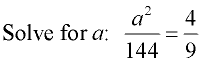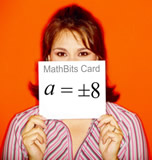Choose: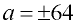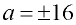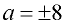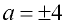2.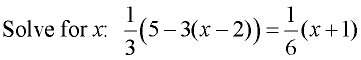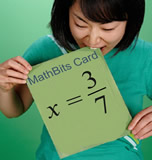Choose: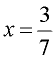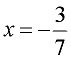x = 3 x = -3

3.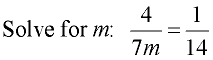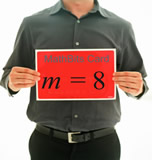Choose: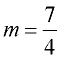m = 2 m = 4 m = 8

4.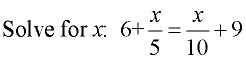Choose:
 x = 10 x = 20 x = 30 x = 40

5.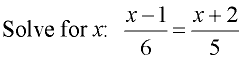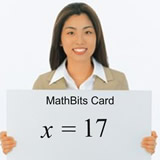Choose:
 x = 7 x = -7 x = 17 x = -17

6.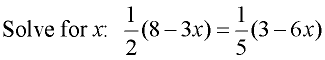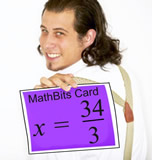Choose: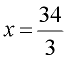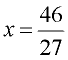11 -11

7.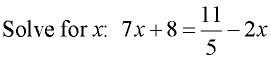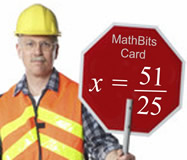Choose: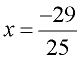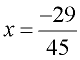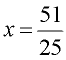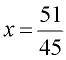8.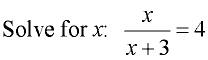Choose: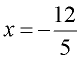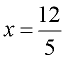x = -4 x = 4

9.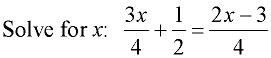Choose:
 x = 1 x = -1 x = 5 x = -5

10.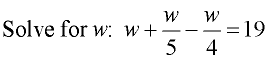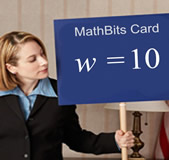Choose:
 w = 1 w = 2 w = 10 w = 20

11.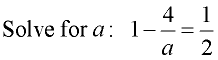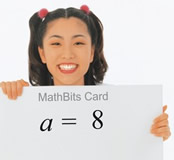Choose:
 a = -4 a = 2 a = 4 a = 8

12.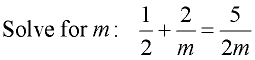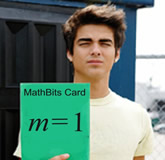Choose:
 m = 1 m = -1 m = 9 m = -9

13.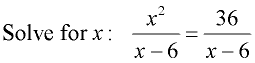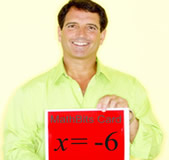Choose:
 x = 6 x = -6 x = ±6 x = ±12

14.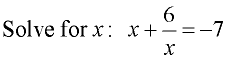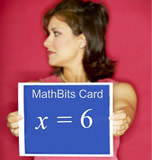Choose:
 x = -1 x = 6 x = -6, -1 x = 1, 6